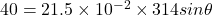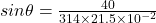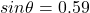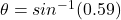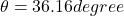## A force of 314 N is exerted on the end of a wrench in order to apply a torque of 40.0 NÂ·m to a bolt head. The point of application of the f

Question

A force of 314 N is exerted on the end of a wrench in order to apply a torque of 40.0 NÂ·m to a bolt head. The point of application of the force is 21.5 cm from the center of the bolt. What angle does the force make with respect to the wrench handle?

in progress 0
1 month 2021-08-16T02:53:18+00:00 2 Answers 0 views 0

Explanation:

Force, F = 314 N

Torque, τ = 40 Nm

distance, r = 21.5 cm = 0.215 m

Let the angle is θ.

τ = r x F x Sin θ

40 = 0.215 x 314 x Sin θ

Sin θ = 0.5925

θ = 36.3°

36.16 degree

Explanation:

We are given that

Force,F=314 N

Torque=T=40 Nm

Distance,r=21.5 cm=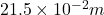1 m=100 cm

We have to find the angle made by force with respect to the wrench handle.

We know that

Torque,T=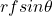Using the formula